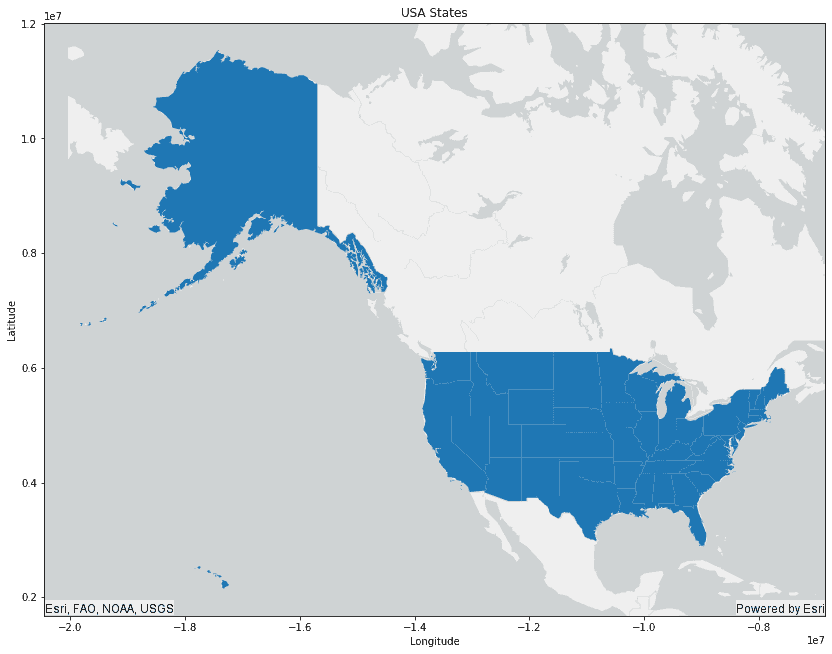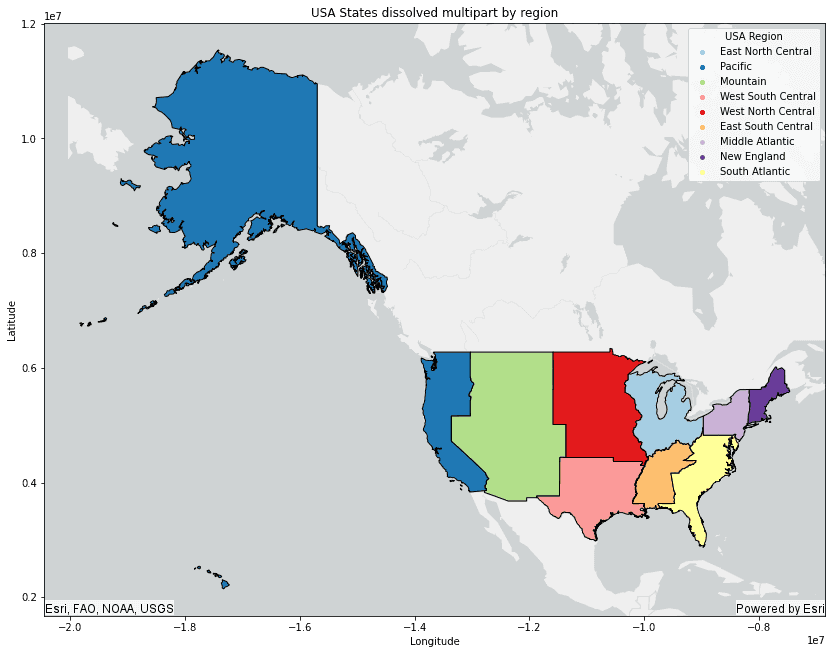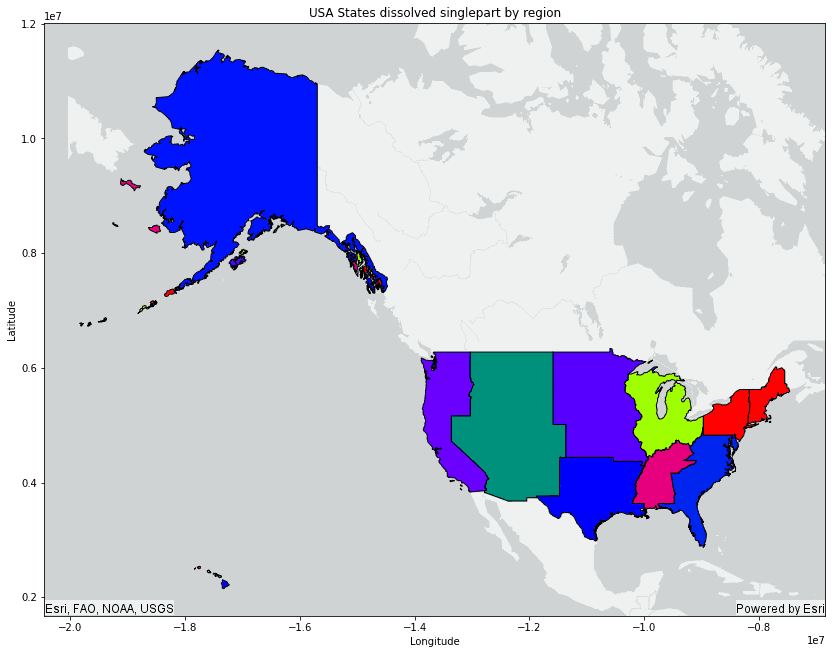# Dissolve Boundaries Workflow

This covers the Spark SQL workflow that replicates the Dissolve Boundaries tool. Dissolve Boundaries merges geometries that intersect or have the same field value into a single geometry. This workflow will dissolve the USA States data by region, calculate the summary statistics for each dissolved region, and convert the dissolved multipart geometries into singlepart geometries.

## Prerequisites

The following are required for this tutorial:

1. A running Spark session configured with ArcGIS GeoAnalytics Engine.
2. A notebook connected to your Spark session (e.g. Jupyter, JupyterLab, Databricks, EMR, etc.).
3. An internet connection (for accessing sample data).

## Steps

### Import and authorize

1. In your notebook, import geoanalytics, the spatial type and PySpark SQL functions and authorize the module using a username and password, or a license file.

Python
Use dark colors for code blocksCopy

``````import geoanalytics
from geoanalytics.sql import functions as ST
from pyspark.sql import functions as F

``````

### Read the sample data and plot

1. Create a DataFrame from a feature service of the state boundaries in the United States and display columns of interest.

Python
Use dark colors for code blocksCopy

``````# Create a DataFrame from the USA States Boundaries feature service
url = "https://services.arcgis.com/P3ePLMYs2RVChkJx/ArcGIS/rest/services/USA_State_Boundaries/FeatureServer/0"

# Display the first 5 rows of the DataFrame
df.select('STATE_NAME', "SUB_REGION", "POP2010").show(5)
``````
Result
Use dark colors for code blocksCopy

``````+----------+----------+--------+
|STATE_NAME|SUB_REGION| POP2010|
+----------+----------+--------+
|California|   Pacific|37253956|
|    Hawaii|   Pacific| 1360301|
|     Idaho|  Mountain| 1567582|
+----------+----------+--------+
only showing top 5 rows``````
2. Plot the USA States data.

Python
Use dark colors for code blocksCopy

``````# Plot the USA States data
df_plot = df.st.plot(figsize=(14, 14), basemap='light')

df_plot.set_title("USA States")
df_plot.set_xlabel("Longitude")
df_plot.set_ylabel("Latitude")
``````### Dissolve States by region

1. Use the `ST_Aggr_Union` Python function to dissolve the States by the `SUB_REGION` field to create multipart geometries.

Python
Use dark colors for code blocksCopy

``````# Dissolve by SUB_REGION and create multipart geometries
df_dissolved_multipart = df.groupBy("SUB_REGION").agg(ST.aggr_union("shape").alias("dissolved_geom_multipart")) \
.withColumn("wkt", ST.as_text("dissolved_geom_multipart"))
df_dissolved_multipart.show(10)
``````
Result
Use dark colors for code blocksCopy

``````+------------------+------------------------+--------------------+
|        SUB_REGION|dissolved_geom_multipart|                 wkt|
+------------------+------------------------+--------------------+
|           Pacific|    {"rings":[[[-1.78...|MULTIPOLYGON (((-...|
|          Mountain|    {"rings":[[[-1.32...|POLYGON ((-1.3263...|
|West South Central|    {"rings":[[[-1.17...|MULTIPOLYGON (((-...|
|West North Central|    {"rings":[[[-1.05...|POLYGON ((-1.0583...|
|East South Central|    {"rings":[[[-9469...|POLYGON ((-946995...|
|       New England|    {"rings":[[[-8185...|MULTIPOLYGON (((-...|
|    South Atlantic|    {"rings":[[[-8993...|MULTIPOLYGON (((-...|
|East North Central|    {"rings":[[[-9804...|MULTIPOLYGON (((-...|
|   Middle Atlantic|    {"rings":[[[-8403...|MULTIPOLYGON (((-...|
+------------------+------------------------+--------------------+``````
2. Plot the dissolved multipart geometries.

Python
Use dark colors for code blocksCopy

``````# Plot the dissolved multipart geometries
df_dissolved_multipart_plot = df_dissolved_multipart.st.plot(cmap_values="SUB_REGION", is_categorical=True, cmap="Paired",
legend=True, legend_kwds={'title':"USA Region"},
figsize=(14, 14), edgecolor="black", basemap="light")

df_dissolved_multipart_plot.set_title("USA States dissolved multipart by region")
df_dissolved_multipart_plot.set_xlabel("Longitude")
df_dissolved_multipart_plot.set_ylabel("Latitude")
``````### Calculate summary statistics for the dissolved regions

A full list of summary statistics can be found in summary statistics.

1. Calculate the total population for each region.

Python
Use dark colors for code blocksCopy

``````# Get the sum of the population for each "SUB_REGION"
df.groupBy("SUB_REGION").sum().select("SUB_REGION", "sum(POP2010)").show(10)
``````
Result
Use dark colors for code blocksCopy

``````+------------------+------------+
|        SUB_REGION|sum(POP2010)|
+------------------+------------+
|           Pacific|    49880102|
|West South Central|    36346202|
|   Middle Atlantic|    40872375|
|    South Atlantic|    59777037|
|East North Central|    46421564|
|       New England|    14444865|
|          Mountain|    22065451|
|East South Central|    18432505|
|West North Central|    20505437|
+------------------+------------+``````
2. Calculate the number of States within each region.

Python
Use dark colors for code blocksCopy

``````# Get the count of States within each "SUB_REGION"
df.groupBy("SUB_REGION").count().select("SUB_REGION", "count").show(10)
``````
Result
Use dark colors for code blocksCopy

``````+------------------+-----+
|        SUB_REGION|count|
+------------------+-----+
|West South Central|    4|
|West North Central|    7|
|    South Atlantic|    9|
|           Pacific|    5|
|       New England|    6|
|          Mountain|    8|
|   Middle Atlantic|    3|
|East South Central|    4|
|East North Central|    5|
+------------------+-----+``````

### Create dissolved singlepart geometries

1. Convert the dissolved multipart geometries into dissolved singlepart geometries using the `ST_Geometries` Python function and the PySpark `Explode` function.

Python
Use dark colors for code blocksCopy

``````# Create dissolved singlepart geometries from the dissolved multipart geometries
df_dissolved_singlepart = df_dissolved_multipart.select("SUB_REGION",
F.explode(ST.geometries("dissolved_geom_multipart")) \
.alias("dissolved_geom_singlepart")) \
.withColumn("wkt", ST.as_text("dissolved_geom_singlepart")) \
.withColumn("index", F.monotonically_increasing_id())

df_dissolved_singlepart.orderBy("SUB_REGION", desc=False).show(20)
``````
Result
Use dark colors for code blocksCopy

``````+------------------+----------------------------+--------------------+-----+
|        SUB_REGION|dissolved_geom_non_multipart|                 wkt|index|
+------------------+----------------------------+--------------------+-----+
|East North Central|        {"rings":[[[-9851...|POLYGON ((-985149...|   79|
|East North Central|        {"rings":[[[-9804...|POLYGON ((-980408...|   77|
|East North Central|        {"rings":[[[-9851...|POLYGON ((-985185...|   80|
|East North Central|        {"rings":[[[-9688...|POLYGON ((-968863...|   78|
|East North Central|        {"rings":[[[-9334...|POLYGON ((-933466...|   81|
|East South Central|        {"rings":[[[-9469...|POLYGON ((-946995...|   57|
|   Middle Atlantic|        {"rings":[[[-8403...|POLYGON ((-840342...|   82|
|   Middle Atlantic|        {"rings":[[[-8264...|POLYGON ((-826401...|   85|
|   Middle Atlantic|        {"rings":[[[-8158...|POLYGON ((-815894...|   84|
|   Middle Atlantic|        {"rings":[[[-8210...|POLYGON ((-821005...|   83|
|          Mountain|        {"rings":[[[-1.32...|POLYGON ((-1.3263...|   46|
|       New England|        {"rings":[[[-7933...|POLYGON ((-793364...|   59|
|       New England|        {"rings":[[[-7859...|POLYGON ((-785963...|   60|
|       New England|        {"rings":[[[-8185...|POLYGON ((-818536...|   58|
|       New England|        {"rings":[[[-7795...|POLYGON ((-779589...|   61|
|       New England|        {"rings":[[[-7612...|POLYGON ((-761290...|   62|
|           Pacific|        {"rings":[[[-1.78...|POLYGON ((-1.7819...|    0|
|           Pacific|        {"rings":[[[-1.77...|POLYGON ((-1.7737...|    1|
|           Pacific|        {"rings":[[[-1.75...|POLYGON ((-1.7552...|    2|
|           Pacific|        {"rings":[[[-1.74...|POLYGON ((-1.7445...|    3|
+------------------+----------------------------+--------------------+-----+
only showing top 20 rows``````
2. Plot the dissolved singlepart geometries.

Python
Use dark colors for code blocksCopy

``````# Plot the dissolved singlepart geometries
df_dissolved_singlepart_plot = df_dissolved_singlepart.st.plot(cmap_values="index",
is_categorical=True,
cmap="prism",
figsize=(14, 14),
edgecolor="black",
basemap="light")

df_dissolved_singlepart_plot.set_title("USA States dissolved singlepart by region")
df_dissolved_singlepart_plot.set_xlabel("Longitude")
df_dissolved_singlepart_plot.set_ylabel("Latitude")``````## What's next?

See below for some related topics: